## Franklin Pezzuti Dyer

### Intransitive random variables

Imagine that a swindler proposes a simple dice game to you, in which there are three dice, each player rolls one of the die, and whoever rolls the highest number is the winner. However, in order to assure you that there isn't any rigged die that rolls higher numbers than the others in most cases, the swindler gives you the opportunity to choose the die you like best before he chooses his.

Perhaps you would suspect some kind of trickery, but if you have the right to pick your die first, do you think the game could be fair, or even in your favor? What about if the three dice are as follows?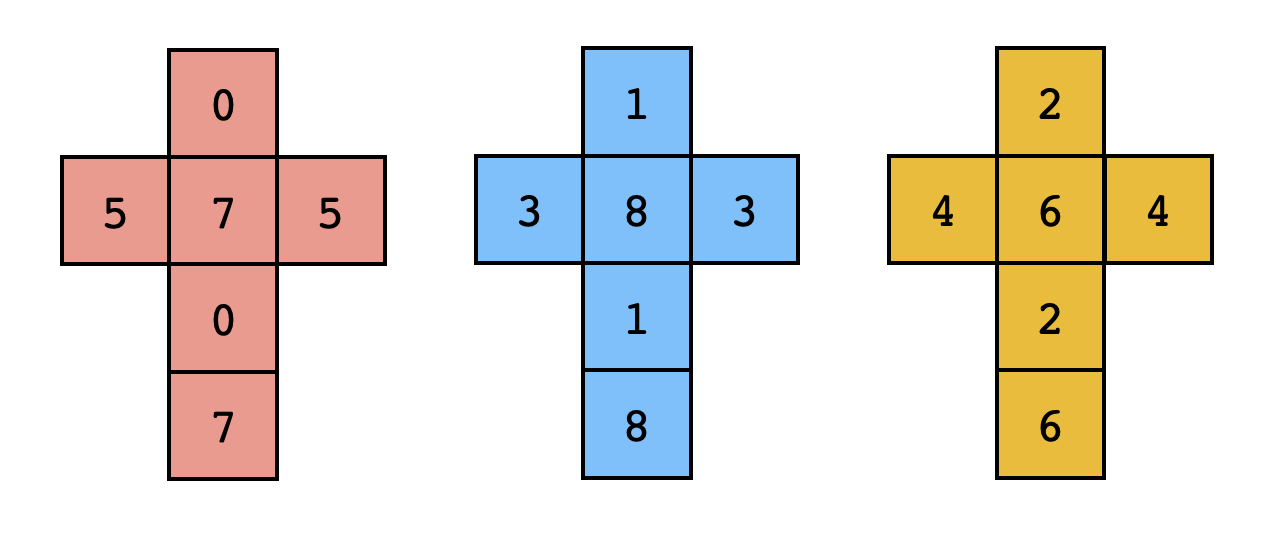These three dice can be described as three random variables:

These three random variables have a very peculiar property - case by case, you can show that

That is, there's no preferable die, but all the same this is no fair game. For each die, there is another die that rolls a higher number than it does in most cases. No matter which die you choose, the swindler can choose another in order to win the game with probability $5/9$.

This phenomenon is called the intransitivity of random variables, and it's sometimes known for its unintuitive nature. In this section I'd like to consider a more general situation, which (in my opinion) sheds a bit of light upon why this phenomenon can seem so perplexing to us.

Imagine for a moment that we're considering three sentences $\psi,\phi,\chi$ that are either true or false for each result of the random variable $W$. That is, if $\Omega$ is the sample space of the random variable $W$, then $\psi,\phi,\chi$ are predicates on the set $\Omega$. If two predicates $\psi,\phi$ are incompatible, or in other words if $\neg(\psi\land\phi)$ is true in each case, then it follows that Now imagine that $\psi,\phi,\chi$ are incompatible, that is, that For instance, if $W$ consists of a triple of random variables $(X,Y,Z)$ then the three sentences are incompatible, although at the moment we're discussing general predicates. Intuitively, it might appear that we could deduce in the same way, and consequently conclude that it's impossible for each of the probabilities $\mathbb P(\psi),\mathbb P(\phi),\mathbb P(\chi)$ to be greater than $1/3$, that is, and that if $\Omega$ were optimally divided up in such a way that maximizes this minimum probability, then it would look something like this: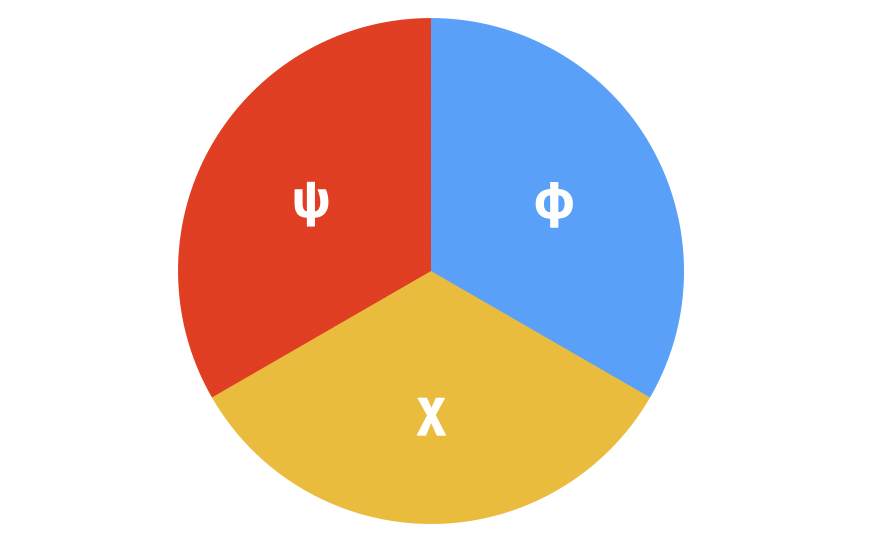This analysis is, however, incorrect, since these three predicates are incompatible but not pairwise incompatible. The statement $\psi\land\phi$ can certainly be true and similarly $\phi\land\chi$ and $\chi\land\psi$ can also be true, even though $\psi\land\phi\land\chi$ is always false. For each result $\omega\in\Omega$, at most two of the three incompatible predicates can be true, and therefore the superposition of the three subsets are capable of covering the sample space $\Omega$ at most twice. Using this observation, we can deduce the following upper bound: and therefore and the limiting distribution would look something like this: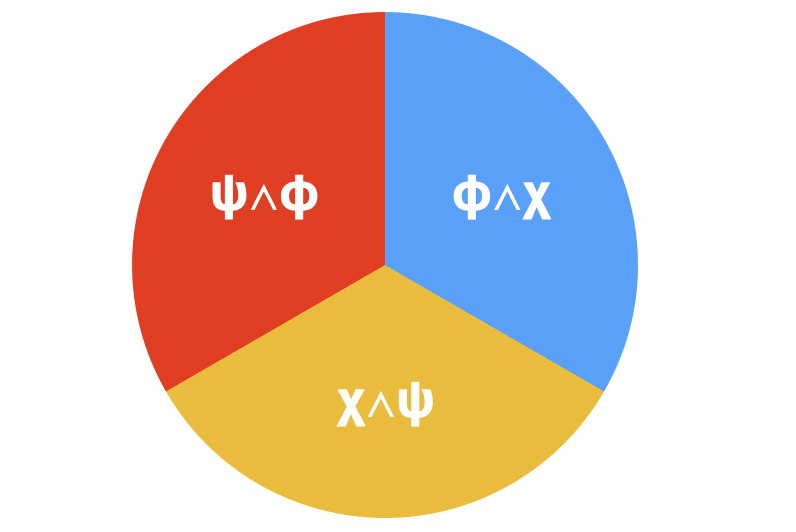In reality there's no paradox here. As far as I can tell, the unintuitive nature of this phenomenon arises from a poor intuition about statement of the form "probably $\psi$". In general, it takes a lot of cognitive effort for us to reconcile the following claims:

• $\psi$ will probably be true.
• $\phi$ will probably be true.
• $\chi$ will probably be true.
• $\psi,\phi,\chi$ will definitely not all be true.

With just two predicates there isn't any "paradox" of this form, since it's not possible for $\psi$ and $\phi$ to both probably be true while $\psi\land\phi$ is impossible, if one interprets "probably $\psi$" as $\mathbb P(\psi) > 1/2$.

#### Maximal intransitivity

We've already seen that with three random variables $X,Y,Z$, there can't be any violation of transitivity worse than the following: Conversely, we can easily confirm that a violation of this magnitude is in fact possible, for instance when $X,Y,Z$ are distributed as follows: But we've left out an important condition on these random variables that would be necessary for them to be considered a realistic generalization of the original dice game, namely that they be independent. The three random variables in the above distribution clearly aren't independent. It could be that we can also find three independent random variables which also attain this upper bound of $2/3$, but we haven't seen any such examples yet.

Imagine a simpler question in which we assume that $Y$ is constant, that is, that there exists a real number $c$ such that $\mathbb P(Y = c) = 1$. We'll also assume that the probability of any two of the random variables being equal is zero. In this case, we'd be looking for the maximal value of the minimum probability if $X,Z$ are independent. It follows, however, that since $X<c$ and $c<Z$ together imply $X < Z$, and these two events are independent (due to this assumption we can multiply their probabilities). Therefore, the desired maximal value is at most However, you can confirm for yourself that this minimum value reaches its peak when $p=q=1-pq$, that is, when Therefore, we know that if $Y$ is constant, the maximum violation of transitivity that we can attain is at most $\phi^{-1}\approx 0.618$, but as for whether this bound is attainable or not, we still don't know. As a matter of fact, from this special case, a more general bound can be derived: in this manuscript by S. Trybula it's proven that the maximal violation of transitivity is $\phi^{-1}$ even when $Y$ is not assumed to be constant. That is:

It's a neat puzzle to try determining whether there exist three independent random variables that reach this upper bound. The fact is that such a triple does exist, and there even exists such a triple in which $Y$ is constant: We could equivalently describe these random variables by means of their probability density distributions: which can be visualized as looking something like this: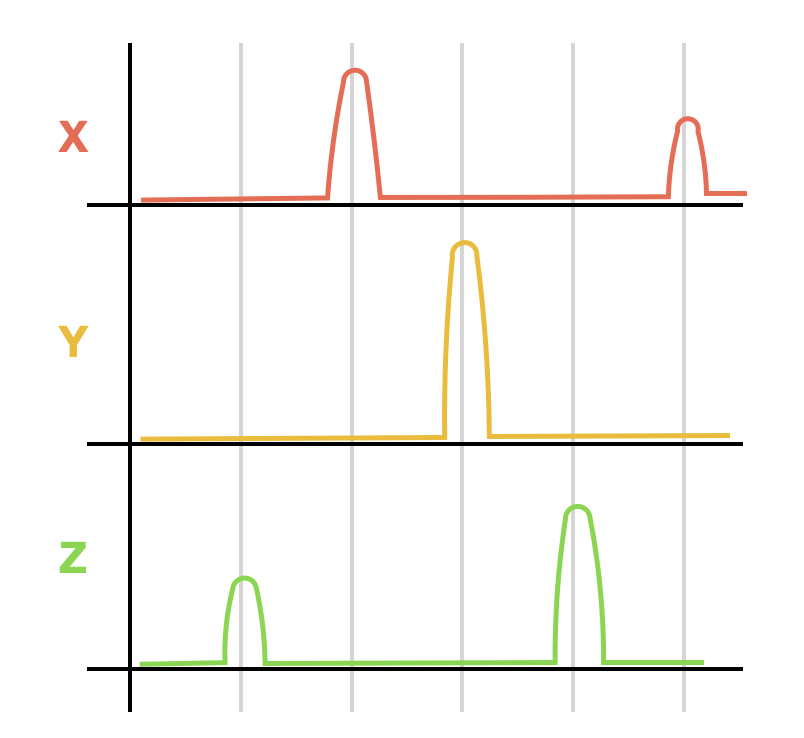When it comes to four or more random variables, I'm not sure what the maximal violation of transitivity would be. With more random variables, the upper bound of $\phi^{-1}$ can be surpassed, but it can be proven without too much difficulty that there's a universal upper bound of $3/4$, that is, if $X_1,X_2,\cdots, X_n$ are independent random variables, then it must be the case that Can you prove this upper bound? If you'd like a hint, I'd suggest that it could be useful to consider medians of these $n$ random variables.

#### A parametrized family

Let's suppose for a moment that we have three random variables $X,Y,Z$ and that we describe a whole family of random variables as follows: where $p+q+r=1$. That is, $W(p,q,r)$ is a random variable that takes its value from $X$ with probability $p$, from $Y$ with probability $q$ and from $Z$ with probability $r$. In other words, if $f_X,f_Y,f_Z$ are the respective probability density distributions of $X,Y,Z$, then the distribution of $W(p,q,r)$ would be described by We could parameterize this family of random variables as three-dimensional vectors from $\mathbb R^3$: If we let the parameters $p,q,r$ take on all possible values from the interval $[0,1]$ such that $p+q+r=1$, then the space of all random variables belonging to this family manifests itself as a triangle in $\mathbb R^3$: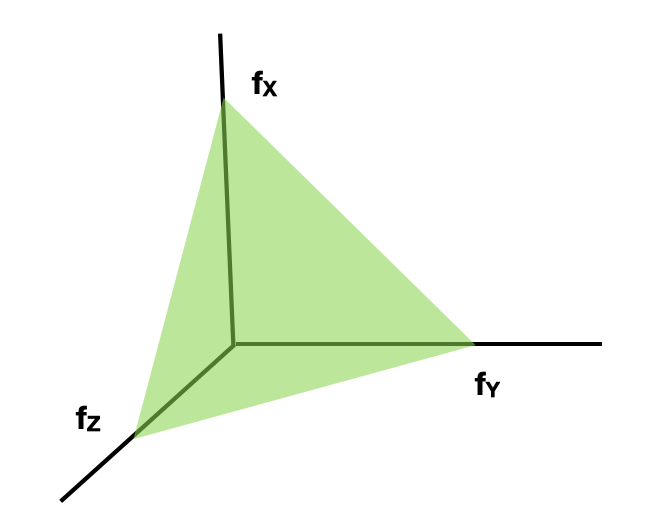To be precise, we're describing an embedding of the convex hull of the three distributions $f_X,f_Y,f_Z$ in the vector space of distributions on $\mathbb R$ in the three-dimensional vector space $\mathbb R^3$ by means of a convex-combination-preserving mapping. Note that the random variables $W(1,0,0), W(0,1,0), W(0,0,1)$ are respectively distributed identically to $X,Y,Z$, and correspond to the three corners of this triangle.

If we assume once more that the three random variables $X,Y,Z$ take on distinct values with probability $1$, and if we know the three probabilities then we can calculate the probability of each possible comparison of random variables from our family $W(p,q,r)$, that is, the probability The parametrization of these random variables as vectors becomes very useful as a way of expressing this probability concisely. If $\mathbf{w}_0, \mathbf{w}_1$ are the vectors corresponding to the respective random variables $W(p_0,q_0,r_0)$ and $W(p_1,q_1,r_1)$, and $A$ is the matrix then one can verify casewise that

Finally, let's consider the case in which $X,Y,Z$ are three independent random variables that maximally violate transitivity. Then we'll have that Notice that we can alternatively describe $A$ as where $C$ is the permutation matrix And this matrix, as a linear transformation on the vector space $\mathbb R^3$, permutes the three coordinates of each vector, that is, it carries out a rotation of $2\pi/3$ radians about the vector $\langle 1,1,1\rangle$: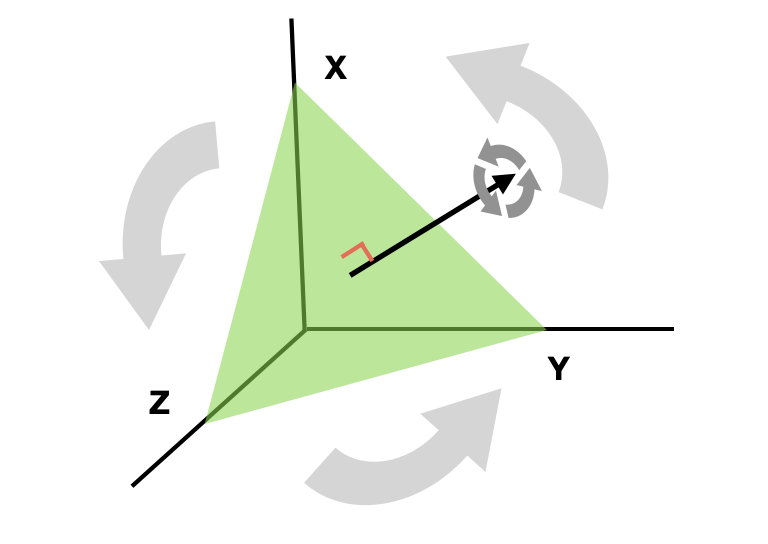The interesting thing is that $C$ is a special case of the more general rotation matrix $R_\theta$ about the vector $\langle 1,1,1\rangle$, so that $C = R_{2\pi/3}$. All rotation matrices about the same axis commute with each other since $R_{\theta_0} R_{\theta_1} = R_{\theta_0 + \theta_1}$, hence we have that for all $\theta$. Consequently, all of the $R_\theta$ commute with $A$, since Hence, the probability of the event $W_0 < W_1$ will stay the same when we rotate the parameter vectors $\mathbf w_0, \mathbf w_1$ by an angle $\theta$ in the plane $x+y+z=1$, that is, replace them with $R_\theta \mathbf w_0, R_\theta \mathbf w_1$, since As a matter of fact, one can calculate the probability $\mathbb P(W_0 < W_1)$ knowing only the respective magnitudes and the angle between the components of the vectors $\mathbf w_0, \mathbf w_1$ in the plane $x+y+z=1$. When their projections onto this plane have magnitudes of $r_0, r_1$ and form a counterclockwise angle of $\Delta\theta$, like this: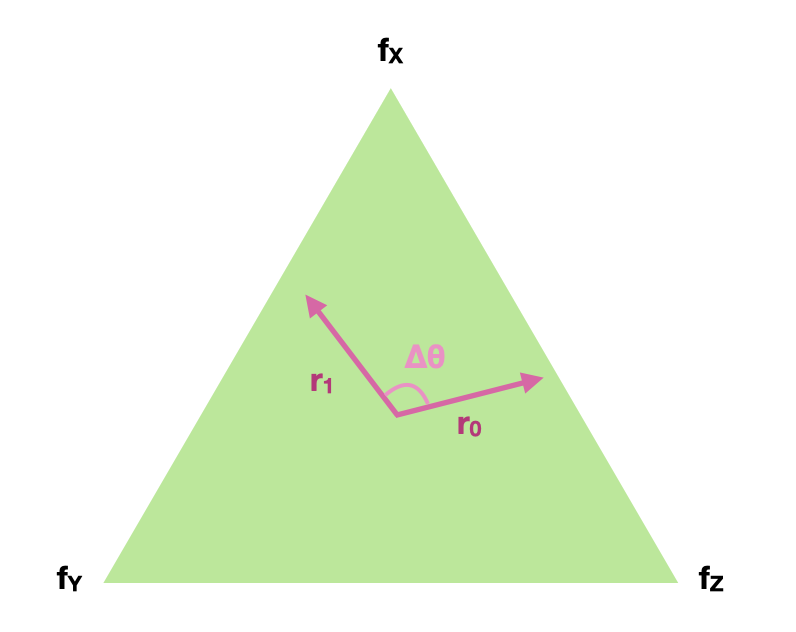then the probability $\mathbb P(W_0 < W_1)$ will be:

Hence, if you want to find a long chain of intransitive random variables $X_1, \cdots, X_n$ so that then it suffices to find $n$ vectors inside of this triangle that have the same length and form congruent angles. For example, if you consider the $5$ random variables corresponding to the vectors or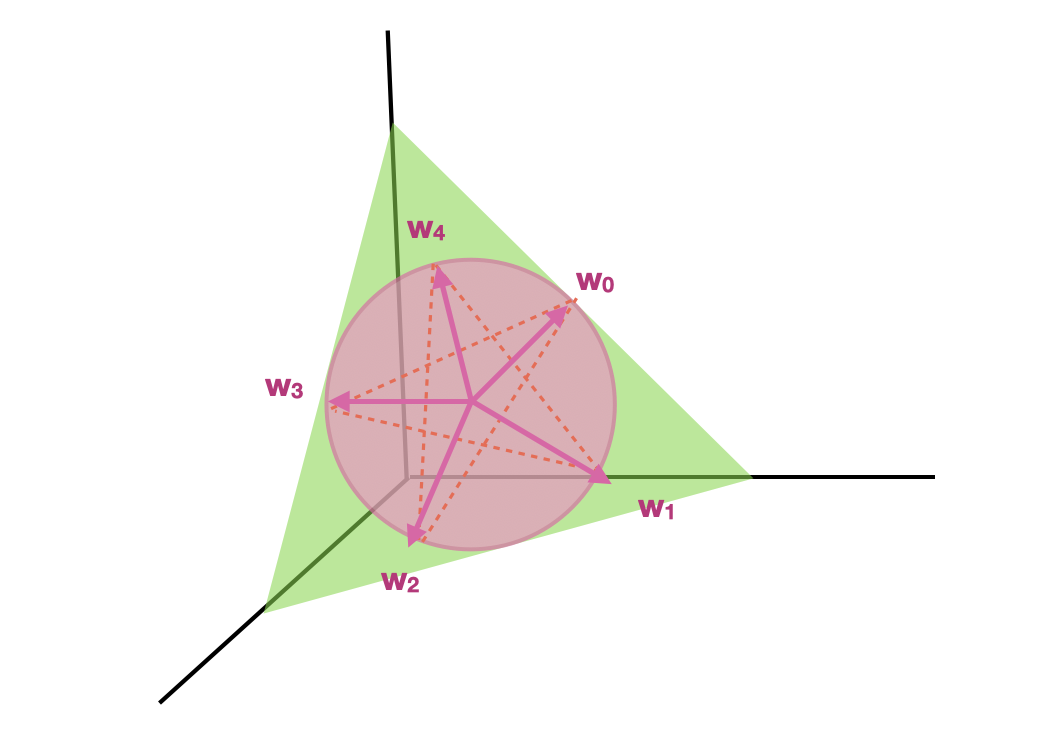then you'll find the (small but non-negligible) transitivity violation of $\approx 0.532$, that is, the minimum of the probabilities $\mathbb P(W_0 < W_1), \cdots, \mathbb P(W_4 < W_0)$ will be $\approx 0.532$. Using this geometric visualization, we can construct larger and more complex families of intransitive random variables, although they won't be optimal in most cases.